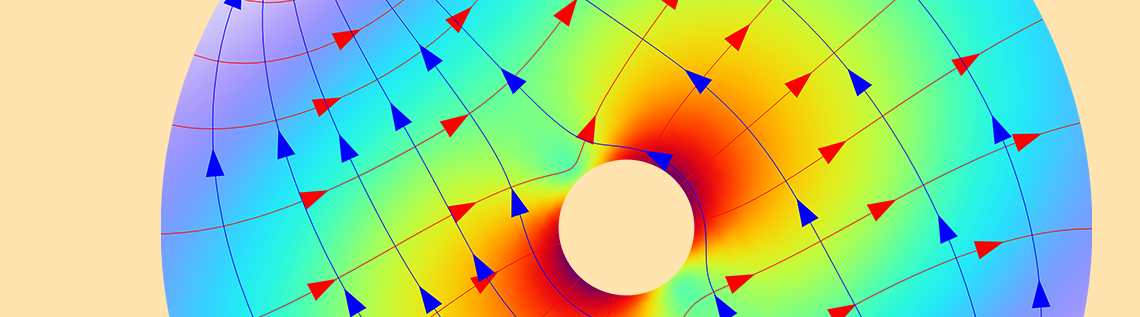# Mode Analysis for Electromagnetic Waveguides in COMSOL®

July 5, 2022

Mode analysis is an essential tool in radiofrequency and wave optics calculations because it allows you to investigate mode characteristics in complex waveguide structures. In this blog post, I will provide an introduction to mode analysis and will summarize the physics interfaces, study steps, and postprocessing settings you will need to carry out this type of study in the COMSOL Multiphysics® software. Then, I will show several examples of pure mode analysis. Lastly, I will explain how to use the results for further calculations of complex RF and optical waveguide systems.

### What Is Mode Analysis?

When analyzing an arbitrary 3D waveguide structure, it is important to understand which types of electromagnetic waves are allowed to propagate at a given frequency. These wave regimes are determined by the resonant modes, which can be excited in a 2D transverse cross section of a waveguide. Such modes can be fully described by the global complex-valued propagation constants and the space distributions of all three components of electric field (so-called mode shapes). Transmission regimes in waveguides with a constant cross section can be defined completely based on these electromagnetic characteristics. We can also use this information for frequency-domain studies of scattering characteristics in more complex structures.

Well-known analytic solutions are only available in the literature for some RF designs, such as coaxial lines and hollow waveguides with rectangular or circular cross sections. For any other configuration with arbitrary shape and material combinations — including all typical optical fibers and integrated waveguides — it is necessary to use numeric mode analysis. Figure 1 shows the formulation of numeric mode analysis in the Equation section of the Settings window. To perform a mode analysis, you will need to plug a given frequency into the Helmholtz equation for electric fields and then search for a solution in the form of a wave traveling in the out-of-plane direction. For that purpose, you can use the finite element method (FEM) and an eigenvalue solver.

Note: Mode analysis should not be confused with the more general modal analysis. The latter is referred to as eigenfrequency analysis and can be used for finding resonant or natural modes and eigenfrequencies in a system of any possible dimension, including 2D, 2D axisymmetric, and 3D.

You can perform mode analysis in the RF Module or Wave Optics Module, both add-on products to COMSOL Multiphysics, by using the following features: the Electromagnetic Waves, Frequency Domain physics interface for 2D or 2D axisymmetric geometry and a Mode Analysis study.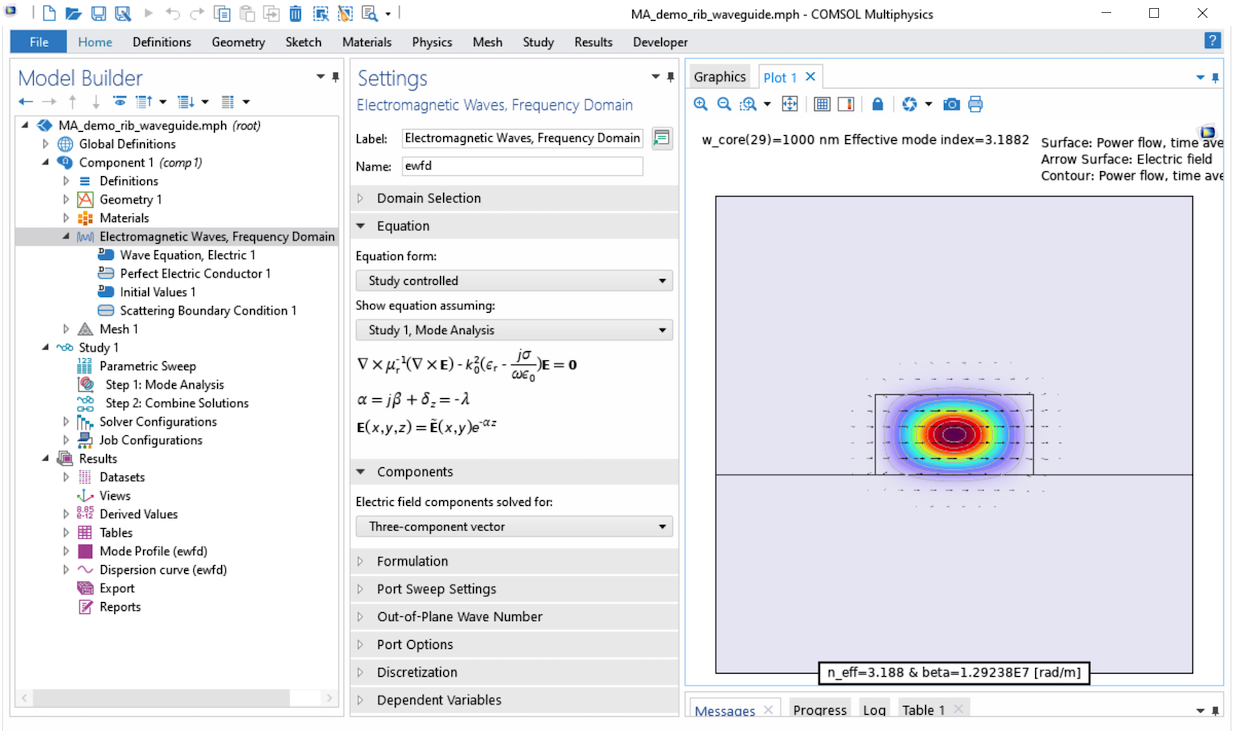Figure 1. Mode analysis for an optical waveguide in COMSOL®. The formulation of such a study is shown in the Equation section of the Settings window for the Electromagnetic Waves, Frequency Domain physics interface.

### How to Set Up Your Model for Mode Analysis

#### Geometry and Material Settings

When setting up a model for mode analysis, you should first build transverse cross sections of a waveguide structure. You can make it directly in 2D or you can reduce a 3D model using the Cross Section operation.

Then, you can specify materials properties and assign them to the corresponding parts of the geometry. For radiofrequency models, you will usually need electrical conductivity, relative permittivity, and relative permeability. For wave optics models, you will usually need a refractive index. COMSOL® automatically converts material data from one representation to another.

Using nonzero conductivity, complex-valued relative permittivity, and complex-valued refractive index will introduce damping in the model, which you can observe in postprocessing.

#### Physics Settings

The goal is to find a wave that is propagating in the out-of-plane direction. To do so using the Electromagnetic Waves, Frequency Domain physics interface in 2D, open the Settings window for the physics interface and make sure the Three-component vector option is selected in the Component section.

Mode analysis is an eigenvalue study, so there is no need to use any source conditions. However, you should still define proper boundary conditions since they will have an affect on mode shape and mode damping and leakage. Note that external boundaries can be metallized or open. If you are working with metallized boundaries, you can use the default Perfect Electric Conductor or Impedance Boundary Condition. In order to describe open boundaries, you can use the Scattering Boundary Condition or a Perfectly Matched Layer.

The default settings for the Scattering Boundary Condition and a Perfectly Matched Layer work well in situations where the electromagnetic wave moves in the normal direction toward the boundary. Such default settings are not optimal for mode analysis since the wave vector of interest is composed of the propagation constant, which is directed tangentially to the boundary, and a remaining normal component. You should manually tweak the settings for the effective wavelength in the Perfectly Matched Layer feature or enable the Subtract propagation constant from material wave number check box in the Mode Analysis section of the Settings window for the Scattering Boundary Condition. You can find detailed instructions on how to do so in the Leaky Modes in a Microstructured Optical Fiber tutorial model’s PDF documentation.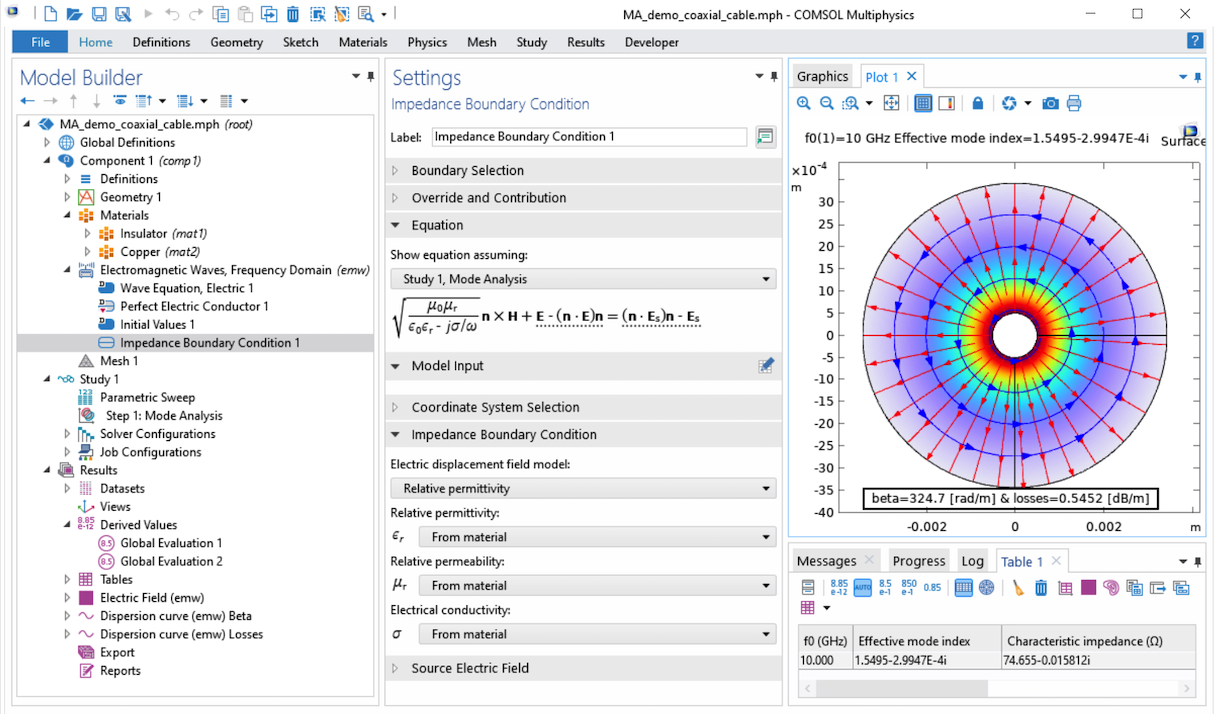Figure 2. Mode analysis for a coaxial cable in COMSOL®. Using the Impedance Boundary Condition allows you to calculate both propagation and attenuation constants.

Using the Impedance Boundary Condition, Scattering Boundary Condition or Perfectly Matched Layer feature will introduce damping into the model.

#### Mesh and Study Settings

A variant of the Mode Analysis study settings for electromagnetics problems is shown in Figure 3 below. By default, the Effective mode index transform is selected, which is usually the best choice for electromagnetic waves. With such a transform, you can assume that the so-called effective index of mode (or effective refractive index) will be used as a declarative characteristic of the mode.

In the Mode analysis frequency field, you should enter the frequency at which you want to find resonant modes. As you can see below, the next study setting listed is Mode search method. If Manual search is selected here, you should set the initial guess in terms of the effective index of mode in the Search for modes around field and the Desired number of modes. The solver will search for modes near that guess and return the expected number of different modes if possible. For the Region search, you should specify the approximate number of modes and the region of the complex effective index.

You might wonder how to make a good initial guess for a successful calculation. It’s actually case-specific, but I have a basis recommendation for you if you work with typical waveguide structures that consist of core and cladding: In this situation, the modes of interest have effective mode indices somewhere between the refractive coefficients of the two materials, and the fundamental mode has the highest index. Therefore, setting the initial guess value to be near the core refractive index in the Search for modes around field guarantees that the solver will find the fundamental mode. In other words, the effective index for the fundamental mode of a typical waveguide structure is close to the refractive index for the domains where the mode energy will be confined.

You can add a Parametric Sweep, select any predefined parameter — such as the geometric size, material property, or frequency — and change its value through a specified range. As a result, you will get what are known as dispersion curves. You can even define cut-off conditions for different modes.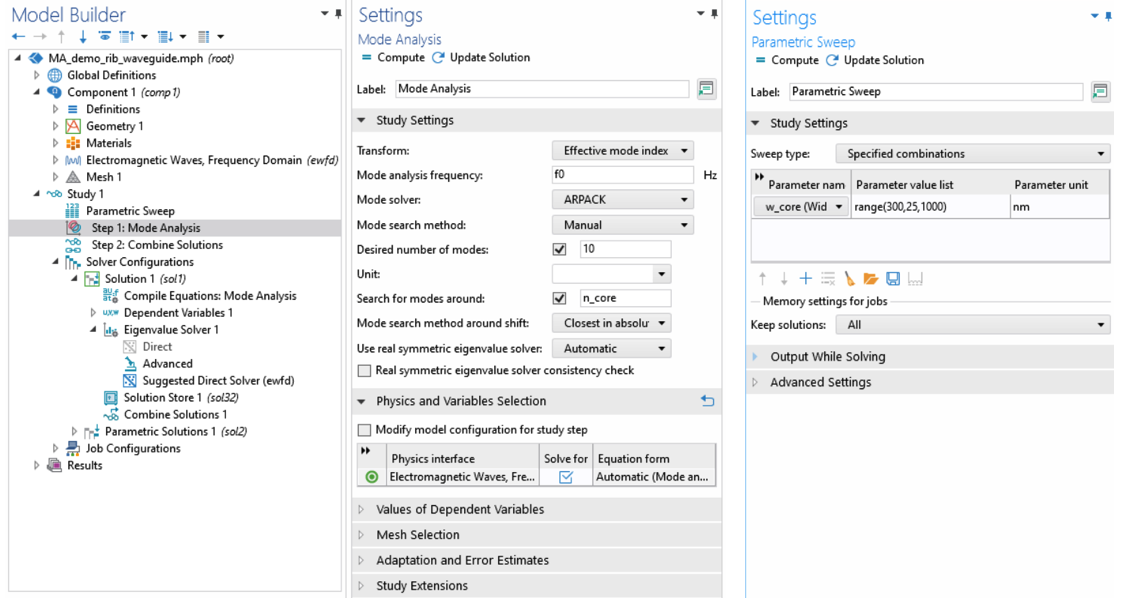Figure 3. Settings for the Modal Analysis and Parametric Sweep study steps. You can see in the expanded Solver Configurations section that an Eigenvalue Solver is used for the study.

While we skipped the mesh settings and chose the study settings first here, note that the entered frequency value in the Mode analysis frequency field is also used for Physics-controlled mesh generation. By default, the software uses five elements per wavelength for each material. For better resolution, you can refine it. For 2D models, such refinement won’t cause any notable memory consumptions.

#### Postprocessing and Results Interpretation

Let’s discuss the typical results that you can get after running the Mode Analysis study.

For each calculated mode, you can plot local field or power flow distributions. You can choose the x-, y-, or z-component or the norm value as an expression. This will allow you to easily define the field profile and polarization. You also have several global variables based on eigenvalue `lambda` for each mode, including the propagation constant, attenuation constant, and effective mode index. You can obtain their exact values via the Global Evaluation feature or using 1D Global plots. The names and definitions of the available variables are summarized in the table below:

Name Expression Description Units
`beta` `imag(-lambda)` Propagation constant rad/m
`dampz` `real(-lambda)` Attenuation constant rad/m
`dampzdB` `20*log10-(exp(1))*dampz` Attenuation in logarithmic scale dB/m
`neff` `j*lambda/k0` Effective mode index 1

For TEM (or quasi-TEM) modes, you can manually calculate the characteristic impedance via the integrals for the current and voltage. The details are discussed in a previous blog post about the usage of numeric ports in RF analyses.

Usually, postprocessing of mode analysis is straightforward, but questions about the correct interpretation of results often come up for the following cases:

• Mode analysis is an eigenvalue study, so exact field amplitude is rather arbitrary and only the relative proportion matters. In postprocessing, you can perform additional normalization for each mode.
• If eigenvalues of two different modes are very close to each other, then you will get any linear combination of them after calculation.
• Calculated modes are just sets of orthogonal solutions that are sorted by the effective mode index. Since the software can’t detect exact physical types of modes, it doesn’t “know”, for example, that the first mode is TE11 and that the sixth mode is TM20. This means that you need to identify the physical type of mode yourself via a manual analysis of field distributions.
• The sorting of modes is performed for each parameter value in a Parametric Sweep, so you can swap the order of modes while moving from one parameter to another. For this, it’s better to plot dispersion curves as a set of points rather than a set of lines.
• Some modes can be unphysical, but you can identify them based on their field profile. In these cases, distributions can be very jagged or can have maxima near the external boundaries.
• Sometimes, modes have a very small propagation constant (close to zero) and a small effective index. You can define them as nonpropagating or evanescent. If you perform a sweep via a relevant control parameter, e.g., frequency, then you can track the cut-off conditions for such a mode.
• Effective mode index can be complex-valued. You can obtain a large imaginary part in two main situations: if the mode is evanescent or if there is some source of damping in the system. Damping can be introduced by a material property, metallization with finite conductivity, and/or an open boundary.

### Tutorial Model Examples of Mode Analysis

Let’s walk through some models that demonstrate two different ways to perform mode analysis in COMSOL®, with extended settings and postprocessing. Below, you can find more information about their setup.

If you would like to jump right into building these models yourself, you can download them here: “Mode Analysis for Electromagnetic Waveguides”.

#### Example 1: Mode Analysis for a Coaxial Cable

Let’s start with an RF example and calculate the modes of a typical coaxial cable. A cable under observation has an inner radius, `r_i` = 0.5 mm; outer radius, `r_o` = 3.43 mm; insulator relative permittivity, ` eps_r` = 2.4; and conductor parts made from copper. Our goal is to define the characteristics of the main TEM-mode and first higher-order TE11-mode in a range of frequencies from 10 to 20 GHz.

Setting up the model is very simple. First, we define the geometry without metal parts. Then, we add the generic Insulator material for a 2D domain. Next, in the Electromagnetic Waves, Frequency Domain interface, we set the Impedance Boundary Condition for external boundaries and assign the build-in Copper material to them. In the Mode Analysis study step, our settings are `f0` for Mode analysis frequency, 2 for Desired number of modes, and `sqrt(eps_r)` for Search for modes around. We also add a Parametric Sweep with ` f0` as the parameter and enter our frequency range with a small step of 0.2 MHz.

Mode analysis provides two solutions for each frequency. We can examine them via space distributions and global variables. From the two solutions, the one that has an effective index closest to the value of `sqrt(eps_r)` ≈ 1.55 is the one that is the TEM-mode. It has a monotonically growing propagation constant and a small attenuation, below 1 dB/m, in our frequency range. Another solution with a smaller index is the TE11-mode. Both its effective index and propagation constant are very small at the beginning of the frequency range; the attenuation constant is very high in contrast. All of these factors indicate that this mode is nonpropagating for the beginning of the frequency range.

Figure 4. The space distributions of several modes for a coaxial cable. Here, we can see the TEM-mode at 10 GHz (upper-left) and at 20 GHz (upper-right) as well as the TE11-mode at 10 GHz (lower-left) and at 20 GHz (lower-right). The surface plot is for the Z-component of power flow, the red streamlines are for the electric field, and the blue are for the magnetic field. Annotations are used for highlighting propagation and attenuation constants. Note that the TE11-mode for 10 GHz has a very small effective index that is an indicator of an evanescent regime.

To define the cut-off frequency, we can create global 1D plots of `emw.beta` and `emw.dampzdB` and use ` f0` as the expression for x-Axis Data. The sharp jump of the TE11 curve that happens around 15.6 GHz is a good estimation of the cut-off condition.

Figure 5. Propagation constant and attenuation constant as functions of frequency for the TEM-mode and the TE11-mode.

For TEM-mode, we can also evaluate characteristic impedance. Values of the characteristic impedance and cut-off frequency for TE11-mode are good verification factors for numeric mode analysis in COMSOL® because we can compare them with well-known analytic expressions.

#### Example 2: Mode Analysis for a Rib Waveguide

Let’s continue with a wave optics example and calculate the modes of a typical integrated waveguide. The rib waveguide under observation has a core made from Silicon, an upper cladding made from SiON, and a lower cladding made from SiO2. The refractive indices of these materials are `n_core` = 3.48, `n_clad_upper` = 1.51, and `n_clad_lower` = 1.44, respectively. Our goal is to define all possible modes at a certain wavelength, `lda0` = 1.55 um; for the fixed core height, `h_core` = 700 nm; and for various values of the core width, ` w_core`.

Like the previous example, setting up the model for this case is also straightforward. First, we define a geometry that contains three domains for the core, the upper cladding, and the lower cladding. Then, we assign them to materials with optic properties (as described in the previous paragraph). Next, in the Electromagnetic Waves, Frequency Domain interface, we set Scattering Boundary Condition for external boundaries and enable the Subtract propagation constant from material wave number check box. In the Mode Analysis study step, our settings are `f0= c_const/lda0` for Mode analysis frequency; 10 for Desired number of modes; and `n_core` for Search for modes around. We also add a Parametric Sweep with ` w_core` as the parameter and vary it from 300 nm to 1000 nm.

Mode analysis returns ten solutions for each frequency, which we can examine via space distributions and global variables. We can use arrows or streamlines to visualize polarization for each mode and define its type, – EyMN or ExMN. The superscript denotes the dominant polarization direction and the first and second subscript variables denote the number of peaks in the x and y direction, respectively. We can also observe high-order modes, like Ey22 and Ey31, for large values of the `w_core` parameter.

Figure 6. Space distributions of several modes for a rib waveguide. In the upper row we can see the Ey11-mode for a core width of 400 nm (left) and the Ex11-mode for a core width of 400 nm (right), while in the lower row we can see the Ex11-mode for a core width of 1000 nm (left) and the Ey21-mode for a core width of 1000 nm (right). The surface plot is for the Z-component of power flow, and the black arrows represent the electric field. Annotations are used for highlighting the effective mode index and propagation constant.

We can also use the global 1D plot of `ewfd.neff` with `w_core*ewfd.k0` as the expression for x-Axis Data to obtain a typical dispersion curve. Using these plots, we can track very complex behavior in a rib waveguide. We can define the exact points where “swaps” of different modes take place; e.g., Ey11 and Ex11 (around `w_core*ewfd.k0` = 2), Ey21 and Ex21 (around ` w_core*ewfd.k0` = 3.5), etc.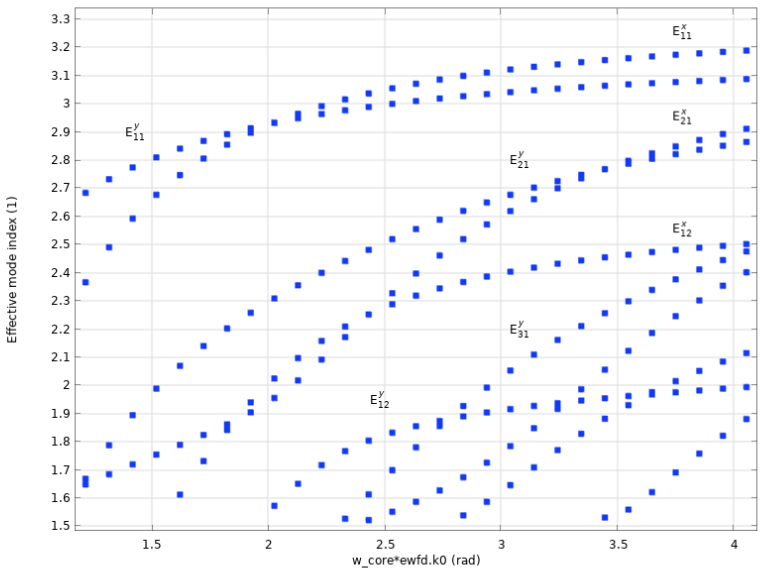Figure 7. Dispersion curves for a rib waveguide. Many mode “swaps” are visible, e.g., there are shifts of Ey11 and Ex11 around `w_core*ewfd.k0` = 2 and of Ey21 and Ex21 around ` w_core*ewfd.k0` = 3.5.

Note that for a small value of core width, we also obtain several modes with an effective index below the refractive index of cladding. Their spatial distribution shows that energy does not confine inside and around the core. We can ignore such modes as unphysical or even remove them via the Remove solution study feature.

#### Other Available Examples

Apart from the two models presented above, I recommend exploring the following examples from the Application Gallery:

### Excitation or Termination of Propagating Modes in Frequency-Domain Waveguide Calculations

Once you have found propagating modes, you may want to excite or terminate one or several of them in a waveguide cross section during a frequency-domain analysis. This will allow you to calculate the reflection and transmission characteristics in a complex electromagnetic device. The capabilities of the RF Module or Wave Optics Module allow you to perform these operations.

For these types of modeling scenarios you can use the Electromagnetic Waves, Frequency Domain or Electromagnetic Waves, Beam Envelopes physics interface. Then, you would typically add the Port boundary condition on each relevant cross section for each relevant mode. After that, you would enable the Numeric option for each Port. For simplicity, let’s call the Port feature with such modifications Numeric Port. For calculation, you could use a combination of the Boundary Mode Analysis for each Numeric Port and Frequency Domain study.

Using a Numeric Port implies that the mode profile and its propagation constant will be calculated during the Boundary Mode Analysis study step, which is almost identical to Mode Analysis. There is one additional Port Name setting where you should specify an exact port name, and the study will perform mode analysis for all the boundaries of this Port. Note that you need to find one exact mode for each port, so you should set the Desired number of modes to 1 and enter a very accurate estimation for the effective mode index in the Search for modes around field. If you do not have any prior knowledge of mode characteristics, it would be a good idea to perform a preliminary mode analysis and define global values for all needed modes — this is especially important when working with the multimode regime. It is also possible to automate a subsequent setup of ports.

This setup is very powerful. First, you can use it directly in 3D without creating a 2D cross section and, moreover, you can use it in 2D for 1D ports. Second, in postprocessing, you receive S-parameters and the reflection and transmission coefficients for a device. Third, you can define a typical wavelength for a Perfectly Matched Layer or specify a phase in the Wave Vector settings of the Electromagnetic Waves, Beam Envelopes physics interface using a propagation constant directly from Boundary Mode Analysis.

Note: For RF devices like microstrip or coplanar lines, there are some special settings for Numeric Port in order to receive TEM or quasi-TEM mode.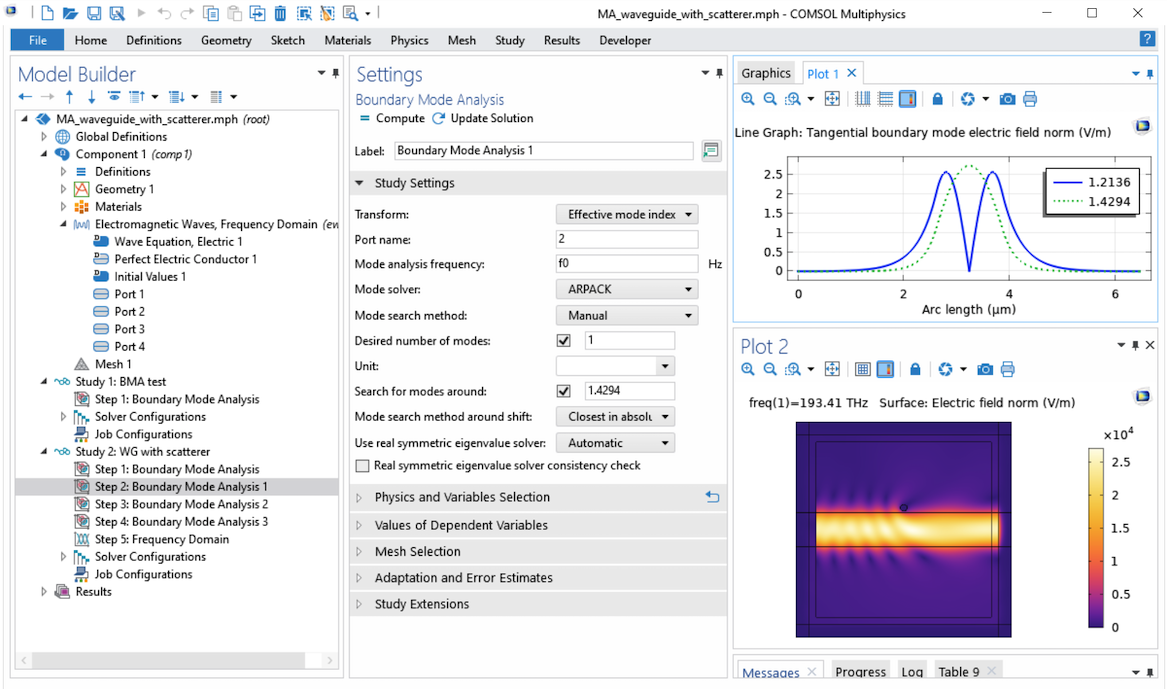Figure 8. The frequency domain analysis of the optic waveguide with a scatterer. Four Numeric Ports are used in this model. You can see the Settings window of the Boundary Mode Analysis for the second Numeric Port, which is used to terminate fundamental mode. Information about its effective index is obtained via a preliminary study.

There are several great examples in the Application Gallery that showcase the use of the Numeric Port conditions and Boundary Mode Analysis studies, such as the:

### Conclusion

In this blog post, we went over how to use the RF Module or Wave Optics Module to find resonant modes in cross sections of waveguide structures and get their qualitative and quantitative characteristics, which can be used in further full-wave studies for the excitation or termination of such modes. In addition, we looked over the typical settings of models with the Mode Analysis study and Numeric Ports. With this information, we can improve the efficiency of our radiofrequency and wave optics calculations.

The techniques discussed here can also be used to investigate wave behavior in acoustics and mechanics applications. For more details, please check out the following models: mode analysis of the muffler cross section with rigid and elastic walls, investigation of elastic wave propagation in a plate, and aeroacoustic calculations of the aero-engine duct.

### Next Step

Try performing mode analysis for a model of a coaxial cable or a rib waveguide yourself using the Mode Analysis for Electromagnetic Waveguides tutorial model:

#### Categories##### Cory Cress
July 19, 2022

Can you explain why you are using w_core*ewfd.k0 as the x-axis to plot the dispersion?##### Sergey Yankin
July 25, 2022 COMSOL Employee

Hi, Cory!

In this case it’s a kind of normalization (in fact w.r.t. wavelength) which is quite useful and popular in optics literature. In your own model you can use any arbitrary expression for x-Axis Data.##### Asma Mimouni
November 9, 2022

Hello,

Thank you for the detailed explanations.
Is it possible to perform a mode analysis of an optical fiber in cylindrical coordinates?

Thank you##### Sergey Yankin
November 9, 2022 COMSOL Employee

Hi, Asma!

Mode analysis is performed in Cartesian coordinates by default. But you can represent your results in cylindrical manner if needed in postprocessing##### Daniel Jedrzejczyk
March 29, 2023

Dear Sergey,

thank you for a very interesting blog post. Is there any particular reason why the fundamental modes in the examples above are labeled with subscript indices of 1? Is this a matter of nomenclature? IMHO these should be labeled with 0 similar to transverse modes of laser beams. In this case the index number corresponds to the number of roots in the transverse electric field distribution.

Kind regards,
Daniel##### Sergey Yankin
April 6, 2023 COMSOL Employee

Hi Daniel!

Are you talking about TE11/Ey21/Ex21 labels? If so, it’s just a common convention for this scientific area.

EXPLORE COMSOL BLOG